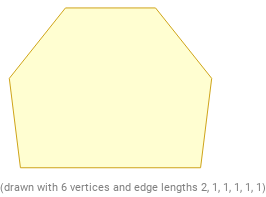Search IntMath
Close

450+ Math Lessons written by Math Professors and Teachers

5 Million+ Students Helped Each Year

1200+ Articles Written by Math Educators and Enthusiasts

Simplifying and Teaching Math for Over 23 Years

# What are Irregular Polygons in Geometry?## Introduction to Polygons

Polygons are two-dimensional shapes with at least three straight sides and angles. Regular polygons have sides that are all the same length and angles that are all the same size. All sides and angles are congruent. Irregular polygons, on the other hand, have sides and angles that are not all congruent. While the terms regular and irregular may sound like they have something to do with the shape of the polygon, they actually have to do with the length of the sides and the size of the angles.

## What is an Irregular Polygon?

An irregular polygon is a polygon that has sides and angles that are not all congruent. Irregular polygons can have any number of sides and angles, and they can be of any shape. Irregular polygons do not have to be symmetrical or regular in shape. The most common example of an irregular polygon is the triangle. A triangle can have three sides of different lengths and three angles of different sizes.

## Properties of Irregular Polygons

Irregular polygons have several interesting properties that can help in solving geometry problems. Some of these properties include:

• The sum of the interior angles is always 180 degrees.
• The sum of the exterior angles is always 360 degrees.
• The sum of the side lengths is always the same, regardless of the shape of the polygon.

## Using Irregular Polygons

Irregular polygons are useful in solving geometry problems involving area, perimeter, and angles. For example, you can use an irregular polygon to calculate the area of a room or the perimeter of a garden. You can also use an irregular polygon to calculate the interior and exterior angles of a shape.

## Practice Problems

Here are some practice problems to help you understand how to use irregular polygons in geometry:

1. What is the sum of the interior angles of an irregular pentagon?
Answer: The sum of the interior angles of an irregular pentagon is 540 degrees.
2. What is the perimeter of an irregular quadrilateral with side lengths of 5, 6, 7, and 8?
Answer: The perimeter of an irregular quadrilateral with side lengths of 5, 6, 7, and 8 is 26.
3. What is the area of an irregular triangle with side lengths of 4, 5, and 6?
Answer: The area of an irregular triangle with side lengths of 4, 5, and 6 is 9.92.
4. What is the sum of the exterior angles of an irregular octagon?
Answer: The sum of the exterior angles of an irregular octagon is 1080 degrees.
5. What is the sum of the side lengths of an irregular heptagon?
Answer: The sum of the side lengths of an irregular heptagon is the same as any other polygon, regardless of the shape.

## Conclusion

In conclusion, irregular polygons are two-dimensional shapes with sides and angles that are not all congruent. Irregular polygons have several useful properties, such as the sum of the interior angles being 180 degrees and the sum of the exterior angles being 360 degrees. Irregular polygons can be used to calculate area, perimeter, and angles in geometry problems.

The key points to remember about irregular polygons in geometry are that they have sides and angles that are not all congruent, the sum of the interior angles is 180 degrees, the sum of the exterior angles is 360 degrees, and they can be used to calculate area, perimeter, and angles.

With practice, you can become a master of irregular polygons and use them to solve geometry problems with ease.

## FAQ

### What is a irregular polygon in geometry?

An irregular polygon is a polygon that does not have all sides and angles equal. It can have any number of sides and angles that are different sizes and shapes.

### What is the best description of an irregular polygon?

An irregular polygon is a polygon with sides and angles that are of different sizes and shapes.

### What is the definition of regular and irregular polygons?

A regular polygon is a polygon with all sides and angles equal, while an irregular polygon is a polygon with sides and angles that are of different sizes and shapes.